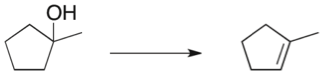# Problem: What would be the optimal conditions to effect the following transformation?  A)              Dilute H2SO4 B)              Concentrated H2SO4 C)              Dilute HBr D)             Concentrated HBr

###### FREE Expert Solution
87% (266 ratings)
###### Problem Details

What would be the optimal conditions to effect the following transformation?

A)              Dilute H2SO4

B)              Concentrated H2SO4

C)              Dilute HBr

D)             Concentrated HBrWhat scientific concept do you need to know in order to solve this problem?

Our tutors have indicated that to solve this problem you will need to apply the Dehydration Reaction concept. You can view video lessons to learn Dehydration Reaction. Or if you need more Dehydration Reaction practice, you can also practice Dehydration Reaction practice problems.

What is the difficulty of this problem?

Our tutors rated the difficulty ofWhat would be the optimal conditions to effect the following...as low difficulty.

How long does this problem take to solve?

Our expert Organic tutor, Jonathan took 1 minute and 3 seconds to solve this problem. You can follow their steps in the video explanation above.

What professor is this problem relevant for?

Based on our data, we think this problem is relevant for Professor Cleveland's class at OLE MISS.